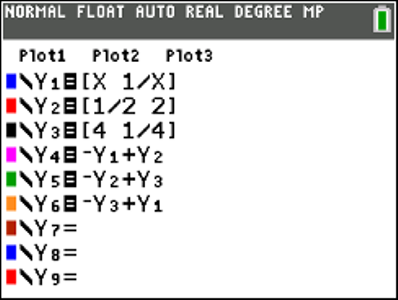•• ##### Device
•TI-84 Plus CE
• ##### Report an Issue

QLD (TI-84PlusCE): Vector Graphics
by Texas Instruments#### Objectives

• Use position vectors to represent points
• Add and subtract vectors in component form
• Determine the dot product of two vectors
• Determine the magnitude of a vector
• Solve problems with vectors

#### Vocabulary

• Component form of a vector
• Magnitude
• Dot Product
• Scalar Product
• Projection

#### About the Lesson

Watch Activity Tutorial

Vectors in component form are used to represent points on the Cartesian plane. Three points in this problem are attached to a the function y = 1/x,. Students use the magnitude of the vectors to compute the perimeter and area of the triangle formed. Finding vectors for the altitudes of the triangle and projections of these vectors using scalar multiples produces an interesting result when students determine the point of intersection of the altitudes.